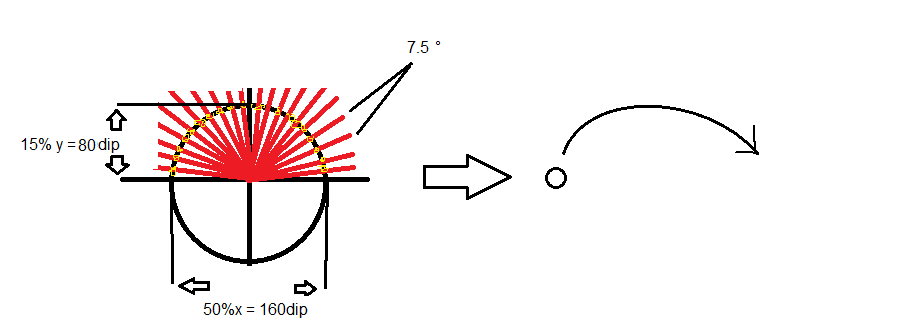# Otherneed some math specialists...

#### ilan

##### Expert
Longtime User
i would like to make jump in a radial path...

i need to calculate the path for example my circle radius is 15% of the screenhight let say (80dip)

and i would like to move the ball from pos x to pos y (50% of the screen widht = 160dip)
and in 24 steps that means 180/24 = 7.5° and i need to get the left and top of the ball view

and add this to a list and then say in a timer ball.left = list1.get(i) and ball.top = list1.get(i2)

how do i calculate the path??

(i need the x/y of the yellow points)Last edited:

#### KMatle

##### Expert
Longtime UserStart in a loop from 0 to 180 (or -90 to +90) step 7.5 (angle you want)

Inside the loop:

x=cos(degree)
y=sin(degree)

I didn't test it but it should work that way. Play with it.

•Erel and ilan

#### derez

##### Expert
Longtime User
B4X:
``````dim a as float
For i = 0 To 24
a = i * 7.5
x = cx - R * CosD(a) - ball.width/2
y = cy - R * SinD(a) - ball.height/2
Next``````

cx , cy - the center of circle, R - the radius.

•KMatle and ilan

#### ilan

##### Expert
Longtime User
thanx i solved it like this

B4X:
``````filljumplist(25%x, 24, "left") 'fill jumping lists jump to left

Sub filljumplist(radius As Int, steps As Double, direction As String)
jumprcalc.Clear

Dim jx As Float
Dim jy As Float
Dim angle As Float = 180/steps
jumpingdir = direction
jumpint = 0

For i = 0 To 24
If direction = "right" Then
Else If direction = "left" Then
End If
'Log(jx & "|" & jy)
Next

End Sub``````

Last edited: